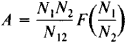devices for measuring the activity—that is, the number of decay events per unit time—of nuclides in radioactive sources. E. Rutherford and H. Geiger may be regarded as the founders of such measurements. In 1903 they carried out the first determination of the specific activity of radium by measuring the number of α-particles emitted in 1 sec by 1 g of the substance. They used a scintillation counter.

Methods of decay measurements can be classified according to the means used for preparing the source, according to the measurement geometry, and according to the physical effects made use of. The first group of methods includes the infinitely thin and infinitely thick layer methods, the tracer transfer into gas method, and the complete sample evaporation method. The second group includes the fixed solid angle method and the 4π counting method. The third group includes the calorimetric, gravimetric, liquid-scintillation-counter, gas-filled-counter, ionization-chamber, mass-spectrometric, emission-spectrum, and coincidence methods.

The 4π counting method is commonly used for absolute measurements of the activity of α- and β-emitters. In this method, the particles emitted by the source in all directions are recorded. The activity is found from the equation

A = N/PK

where N is the counting rate corrected for background and dead time, P is the correction for the decay scheme, and K is a coefficient that takes into account such factors as the absorption in the substrate and the self-absorption in the source. Gas-discharge 4π counters are used for measuring solid radioactive sources. Measurement geometries close to 4π are achieved in the use of liquid scintillation counters and gas-filled counters and chambers.

The coincidence method is used for absolute measurements of the activity of nuclides whose decay is accompanied by cascade emission. The apparatus includes two detectors and is constructed in such a way that different types of radiation or radiations of different energies are measured separately. The technique measures the activity of a source with a nuclide whose decay is accompanied by the cascade emission of precisely these radiations. The activity is determined from the equationwhere N1 and N2 are the count rates obtained with each of the detectors, N12 is the coincidence counting rate, and F is some function of (N1/N2) that tends to unity as (N2/N1) → 1. In the simplest cases, F(N1/N12) = 1.

The calorimetric method is based on the measurement of the thermal effect resulting from the decay of the nuclide in the sample. It is used if the sources have appreciable activity. If we know the mean energy absorbed in the sample-calorimeter system in one decay event and the total intensity of the energy liberated by the source, we can calculate the activity of the nuclides. Although it is one of the oldest methods, the calorimetric method is still widely used today.

If a nuclide can be isolated in macroscopic amounts, its activity may be found from the equation

A = λM

where M is the number of atoms of the nuclide in the sample and λ is the decay constant (in sec–1). This technique is known as the gravimetric method because M is calculated on the basis of the weight of the nuclides in the source. The gravimetric method is called the mass-spectrometric method or the method of emission-spectrum analysis if the relative content of the nuclide in the source is determined by means of mass-spectrometric or emission-spectrum analysis, respectively.

Large-scale measurements of activity are accomplished mainly by comparison methods, wherein the sources being measured are compared with standard sources—very accurately calibrated radioactive solutions, liquids, or gases, whose preparation involves the use of absolute methods for measuring activity. Comparison measurements of the activity of nuclides whose decay is accompanied by γ-radiation are usually carried out by means of ionization chambers, scintillation counters, and semiconductor detectors. In the case of β-emitting nuclides, ionization chambers and gas-discharge counters are used. Large-scale measurements of the activity of low-energy β-emitters, such as 14C and 3H, are carried out with liquid scintillation counters.

Radioactive decay measurements are used in solving a variety of problems. They find application, for example, in research with tracer atoms (seeISOTOPE TRACERS), in the determination of the age of rocks (seeGEOCHRONOLOGY), and in archaeology.

### REFERENCES

Karavaev, F. M. Izmereniia aktivnosti nuklidov. Moscow, 1972.
Korobkov, V. I., and V. B. Luk’ianov. Melody prigotovleniia preparatov i obrabotki rezul’tatov izmerenii radioaktivnosti. Moscow, 1973.
Turkin, A. D. Dozimetriia radioaktivnykh gazov. Moscow, 1973.
Wang, C, and D. Willis. Radioindikatornyi metod v biologii. Moscow, 1969. (Translated from English.)
Tekhnika izmerenii radioaktivnykh preparatov: Sb. st. Moscow, 1962.
Mann, W. B., and H. H. Seliger. Prigotovlenie i primenenie etalonnykh radioaktivnykh preparatov. Moscow, 1960. (Translated from German.)

V. A. BAZHENOV

Site: Follow: Share:
Open / Close